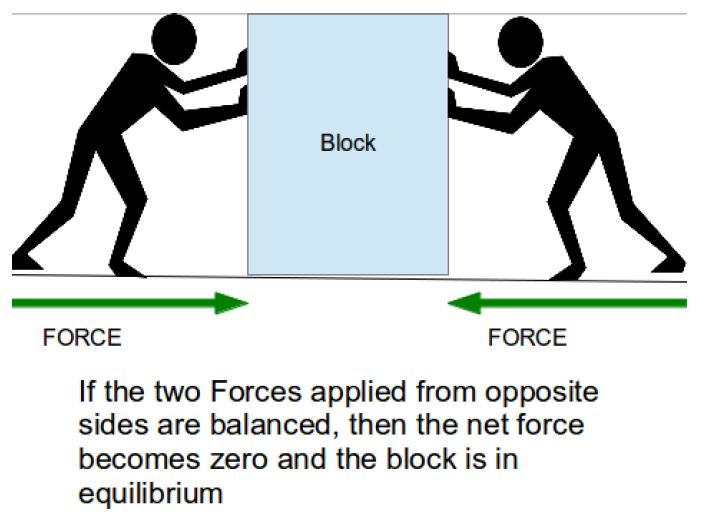# Newton’s 1st Law of MotionSir Isaac Newton was one of the greatest physicist and responsible for development of laws of motion, laws of gravitational attraction and calculus among other things.

Statement of the First law of Motion: An object at rest will remain at rest, and an object in motion will remain in motion at constant velocity and in a straight line, unless acted upon by a net force.

The first law of motion is often called the Law of Inertia.

Let us discuss a few terms and phrases from the statement so that we can understand it better.

Object at rest – Very simply a stationary object (also denoted as static). Objects which are stationary will remain stationary unless there is some force acting on it. This is very intuitive and easy to understand.

Object in motion at constant velocity and in a straight line – This means that the object has constant velocity in moves in some particular direction along a straight line.

The only reason such objects will change its speed or direction of movement, is if an external net force acts on it.

Imagine a rock floating in space in a vacuum. Unless there is some force pulling or pushing it back, forward or sideways – the rock will continue moving in a straight line with a constant velocity.

Net force – We know that force is a push or a pull in some direction. Since force is a vector, we can say that if the vector sum of all forces on the object is non-zero, then there is a net force. Thus we have a net force on a body arising due to unbalanced forces.

Only an unbalanced force will cause an object to deviate from its static state or state of motion with constant velocity along a straight path.

If the vector sum of the forces adds up to cancel each other, then there is no net force on the body.

Thus now we have a good intuitive idea of what the first law of motion is about.

Let us now discuss some very important concepts that surround the first law.

## Force

In the realm of the physics as of now, we have discovered four fundamental forces – Gravitational force, Electromagnetic force, Strong nuclear force and Weak nuclear force.

But in our current discussion we are more interested in forces that we encounter in our macroscopic world regularly. So we will avoid discussion about the fundamental forces and their characteristics. Most of the forces we deal with regularly are either electromagnetic or gravitational.

We can for our ease classify them as field forces or contact forces.

Field force – these are forces that can act from a distance. Like gravitational force, electrostatic force (like charges repel and unlike charges attract) or magnetic force (like poles repel while unlike poles attract).

Contact force – contact forces affect objects only when the object is in contact with the source of the force. For example frictional forces or when you push or pull an object with your hands.

The unit used for force is Newtons – denoted with the letter N

As we already discussed above, force is a vector quantity. When we vectorially add up all the forces acting on a body we get the net force.Equilibrium – If the net force turns out to be zero, then the body is said to be in equilibrium or specifically translational equilibrium.

This equilibrium could be static equilibrium or dynamic equilibrium.

Static equilibrium – when the object is at rest , it is in static equilibrium.

Dynamic equilibrium – when the object is moving in a straight line with constant velocity, it is dynamic equilibrium.

Because of the existence of friction, dynamic equilibrium could be a little difficult to imagine intuitively on earth and examples of it could be rare.

## Inertia

Let us now discuss inertia.

Inertia is the tendency of a body or an object to resist a change in velocity.

Inertia depends on the inertial mass of an object.

Suppose two blocks are kept still on a friction-less surface. One of them is very light and small, and the other one is extremely heavy. If we had to push and give them some velocity, intuition tells us that the lighter box would be easier to maneuver than the heavier one.

This is due to inertial mass.

The more inertial mass an object has, the more it will resist to change in velocity.

Mass is classified as:

• Inertial mass

Inertial mass is responsible for inertia of an object, i.e. the tendency of a body or an object to resist a change in velocity.

Inertia and inertial mass of an object are used interchangeably.

• Gravitational mass

Gravitational mass affects the gravitational force felt by an object when it is in under the influence of a gravitational field.

For all practical purposes, gravitational mass and inertial mass are same.

## Example

Suppose we have huge stationary boulder with mass over a 1000kg in the middle of football pitch and a football weighing less than a kilogram, which has been kicked and hence is travelling with a high velocity. Which has a higher inertia?

We must remember inertia only depends on mass of an object. So the boulder definitely has higher inertia.

[box type=”success” align=”” class=”” width=””]Newton’s 1st Law of Motion is discussed and explained very thoroughly by Prof. Dan Fullerton, in his lecture video on First Law of Motion.[/box]

SPECIAL OFFER!

SUCCESS8